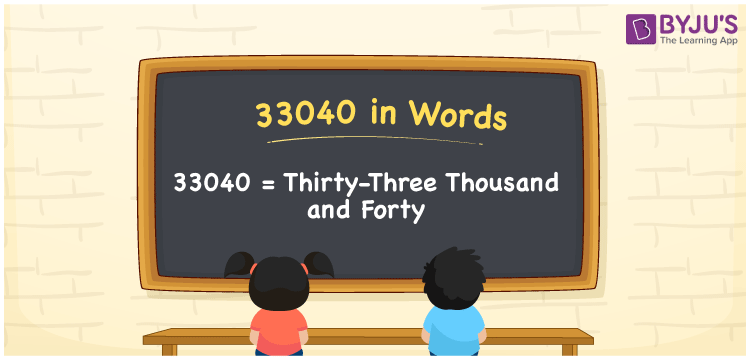# 33040 in Words

The number name of 33040 is Thirty-three thousand and forty. A number 33040 is a cardinal number as it represents the count of the objects or expresses the values. For converting the number 33040 into words, the place value table is very helpful. Here, we will discuss how to write 33040 in words with its procedure in detail.

 33040 in Words: Thirty-three Thousand and Forty. Thirty-three Thousand and Forty in Numerical Form: 33040.

## 33040 in English Words## How to Write 33040 in Words?

The number 33040 can be converted into words, using the place value system. The following is the place value table for the number 33040.

 Ten-thousands Thousands Hundreds Tens Ones 3 3 0 4 0

Thus, the expanded form for the number 33040 is as follows:

= 3 × Ten thousand + 3 × Thousand + 0 × Hundred + 4 × Ten + 0 × One

= 3 × 10000 + 3 × 1000 + 4 × 10

= 30000 + 3000 + 40

= 33040

= Thirty-three thousand and forty

Therefore, 33040 in words is thirty-three thousand and forty.

33040 in words – Thirty-three thousand and forty

Is 33040 an odd number? – No

Is 33040 an even number? – Yes

Is 33040 a perfect square number? – No

Is 33040 a perfect cube number? – No

Is 33040 a prime number? – No

Is 33040 a composite number? – Yes

## Frequently Asked Questions on 33040 in Words

Q1

### How to write 33040 in English words?

33040 in words is thirty-three thousand and forty.

Q2

### Simplify 33000 + 40, and express in words.

Simplifying 33000 + 40, we get 33040. Hence, 33040 in words is thirty-three thousand and forty.

Q3

### Is 33040 a prime number?

No, 33040 is not a prime number.